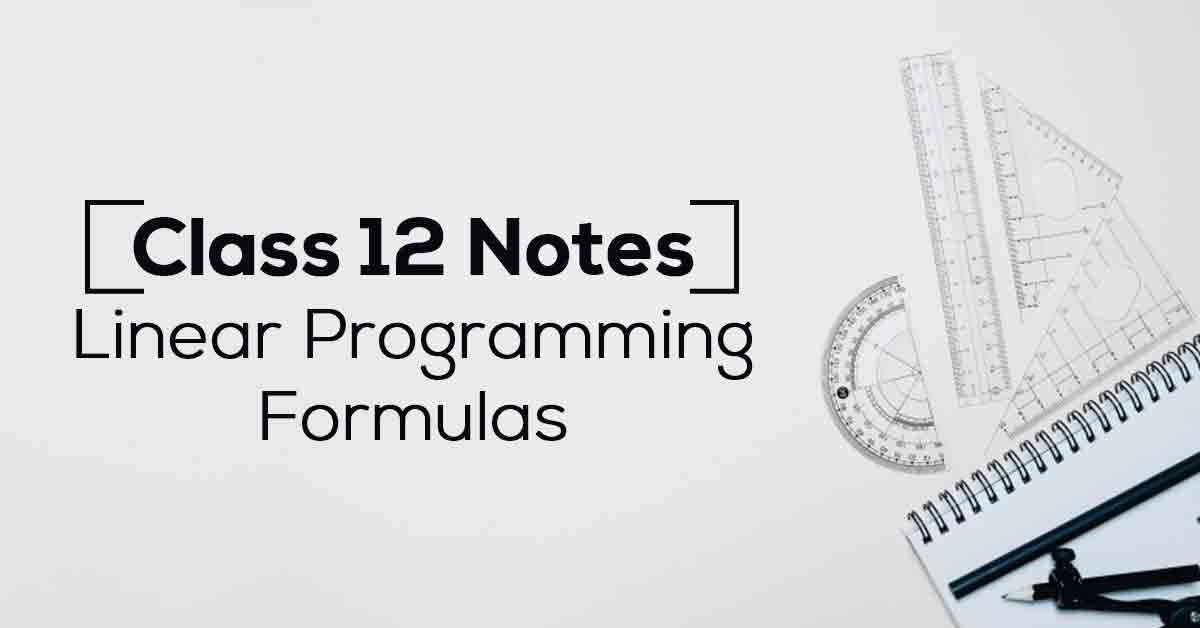Class 12th Math Liner Programming Formulas CBSE 2023

# Linear Programming Class 12 NotesLinear programming is often used in business to find maximum profit or minimum cost. The first step in solving linear programming problems is to set up a function that represents cost, profit, or some other quantity to be maximized or minimized subject to the constraints of the problem. Know More about these in Linear Programming Class 12 Notes List.

The topics and sub-topics covered in Linear Programming Class 12 Notes are:

12.1 Introduction

12.2 Linear Programming Problem and its Mathematical Formulation

12.2.1 Mathematical formulation of the problem

12.2.2 Graphical method of solving linear programming problems

12.3 Different Types of Linear Programming Problems.